# Taylor's Series

Also found in: Dictionary, Wikipedia.
Related to Taylor's Series: Maclaurin series
The following article is from The Great Soviet Encyclopedia (1979). It might be outdated or ideologically biased.

## Taylor’s Series

(or Taylor series), a power series of the form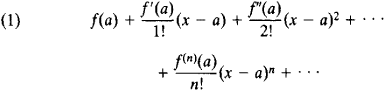where f(x) is a function having derivatives of all orders at x = a.

In many cases of practical importance, Taylor’s series converges to f(x) on some interval with center at a: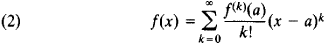(this formula was published in 1715 by B. Taylor). The difference Rn(x) = f(x) – Sn(x), where Sn(x) is the sum of the first n + 1 terms of series (1), is called the remainder. Formula (2) is valid if Rn(x)→ 0 as n→ ∞. A Taylor’s series can be represented in the form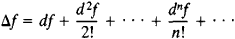which is also applicable to functions of several variables.

When a = 0, the expansion of a function in a Taylor’s series assumes the form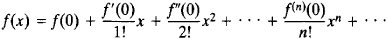Such a series has been traditionally, although incorrectly, called a Maclaurin’s series. Examples of Maclaurin’s series are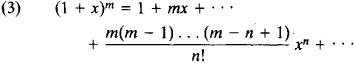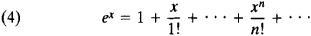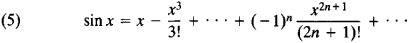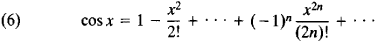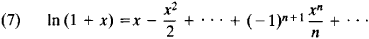Series (3) is a generalization of Newton’s binomial formula to the case where the exponents may be fractional or negative. This series converges for –1 < x < 1 if m < –1, for –1 < x ≤ 1 if – 1 < m < 0, and for – 1 ≤ x ≤ 1 if m > 0. Series (4), (5), and (6) converge for any value of x. Series (7) converges for –1 < x ≤ 1.

If a function f (z) of a complex variable z is regular at the point a, the function can be expanded in a Taylor’s series in powers of z – a within a circle having a center at a and a radius equal to the distance from a to the nearest singular point of f (z). Outside this circle, the Taylor’s series diverges; its behavior on the circumference of the circle of convergence may be very complicated. The radius of the circle of convergence is expressed in terms of the coefficients of the Taylor’s series (seeRADIUS OF CONVERGENCE).

Taylor’s series are used in the study of functions and in approximate calculations (see also).

### REFERENCES

Khinchin, A. Ia. Kratkii kurs matematicheskogo analiza. Moscow, 1953.
Fikhtengol’ts, G. M. Kurs differentsial’nogo i integral’nogo ischisleniia, 7th ed., vol. 2. Moscow, 1969.
References in periodicals archive ?
The Taylor's series expansions of [[summation].sup.p.sub.i=1][w.sub.i]f'([x.sub.n][[tau].sub.i]([z.sub.n] - [x.sub.n])) and [[summation].sup.p.sub.i=1][w.sub.i]f"([x.sub.n] + [[tau].sub.i]([z.sub.n] - [x.sub.n])) are given by
Thus, the analysis of inhomogeneous media using Taylor's series expansion is presented here.
The expression (17) that shows a linear relationship between [E.sup.*] and [alpha], as derived elsewhere [25,26], uses only the first two terms of the Taylor's series expansion for (10).
Expressing the bottom wall velocity using Taylor's series expansion we get,
The figure 24 shows the Taylor's series with two and three terms.
Expanding f ([y.sub.n]) by Taylor's series about w, we have
Some probabilistic methods include Haldar and Tang (1979) and the Taylor's Series reliability method, Wolf (1994); USACE (1997, 1998); Duncan (2000).
In Chapter 4, the finite-difference method for approximating first- and second-order derivatives is derived from Taylor's series. Chapter 5 introduces the Yee cell grid and includes the FDTD expressions for uniform and non-uniform formulations in both homogeneous and inhomogeneous media.
Taylor's series of [W.sub.k] with respect to the random variables is obtained by
"And Peter Taylor's series Brits and Provos were very well received.
Buz'Gem Blues is the third in Taylor's series of blues plays, following Bootlegger Blues and Baby Blues.
Taylor's series Brits, screened tonight on BBC2, includes an interview with one former member of 14 Intelligence who described the cake parties.

Site: Follow: Share:
Open / Close